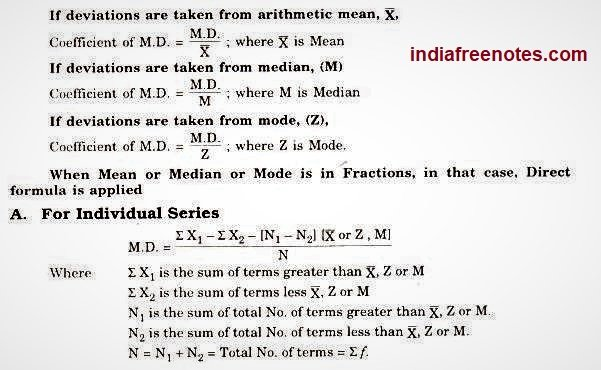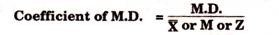# Mean deviation with mean, Co-efficient of mean deviation

18/04/2020

To understand the dispersion of data from a measure of central tendency, we can use mean deviation. It comes as an improvement over the range. It basically measures the deviations from a value. This value is generally mean or median. Hence although mean deviation about mode can be calculated, mean deviation about mean and median are frequently used.

Note that the deviation of an observation from a value a is d= x-aTo find out mean deviation we need to take the mean of these deviations. However, when this value of a is taken as mean, the deviations are both negative and positive since it is the central value.

This further means that when we sum up these deviations to find out their average, the sum essentially vanishes. Thus to resolve this problem we use absolute values or the magnitude of deviation. The basic formula for finding out mean deviation is :

Mean deviation= Sum of absolute values of deviations from ‘a’ ÷ The number of observations

Coefficient of Mean Deviation:

It is calculated to compare the data of two series. The coefficient of mean deviation is calculated by dividing mean deviation by the average. If deviations are taken from mean, we divide it by mean, if the deviations are taken from median, then it is divided by mode and if the “deviations are taken from median, then we divide mean deviation by median.1. For Discrete Series:

M.D. = ∑fdy/N; Where; N=∑f

And dy is the deviation of variable from X, M or Z ignoring signs (Taking +ive signs only).

Steps to Calculate:

1. Take X, M or Z series as desired.
2. Take deviations ignoring signs.
3. Multiply dy by respective f; get ∑fdy
4. Use the following formula

M.D. = ∑fdy/N(Note : If value of X or M or Z is in decimal fractions better use Direct Method to get result easily)

When Mean or Median or Mode is in Fractions, in that case, Direct formula is applied

1. For Continuous Series:

For Continuous Series also ;

M.D. = fdy/N